Multiplication MethodsCustom SearchMULTIPLICATION METHODS The multiplication of whole numbers may be thought of as a short process of adding equal numbers. For example, 6(5) and 6 x 5 are read as six 5’s. Of course we could write 5 six times and add, but if we learn that the result is 30 we can save time. Although the concept of adding equal numbers is quite adequate in explaining multiplication of whole numbers, it is only a special case of a more general definition, which will be explained later in multiplication involving fractions. Grouping Let us examine the process involved in multiplying 6 times 2’7 to get the product 162. We first arrange the factors in the following manner:The thought process is as follows: 1. 6 times ‘7 is 42. Write down the 2 and carry the 4. 2. 6 times 2 is 12. Add the 4 that was carried over from step 1 and write the result, 16, beside the 2 that was written in step 1.  3. The final answer is 162.  Table 2- 1 shows that the factors were grouped in units, tens, etc. The multiplication was done in three steps: Six times 7 units is 42 units (or 4 tens and 2 units) and six times 2 tens is 12 tens (or 1 hundred and 2 tens). Then the tens were added and the product was written as 162. Table 2-l.-Multiplying by a one-digit number.In preparing numbers for multiplication as in table 2- 1, it is important to place the digits of the factors in the proper columns; that is, units must be placed in the units column, tens in tens column, and hundreds in hundreds column. Notice that it is not necessary to write the zero in the case of 12 tens (120) since the 1 and 2 are written in the proper columns. In practice, the addition is done mentally, and just the product is written without the intervening steps. Multiplying a number with more than two digits by a one-digit number, as shown in table 2-2, involves no new ideas. Three times 6 units is 18 units (1 ten and 8 units), 3 times 0 tens is 0, and 3 times 4 hundreds is 12 hundreds (1 thousand and 2 hundreds). Notice that it is not necessary to write the O’s resulting from the step "3 times 0 tens is 0." The two terminal O’s of the number 1,200 are also omitted, since the 1 and the 2 are placed in their correct columns by the position of the 4. Table 2-2.-Multiplying a three-digit number by a one-digit number.Partial Products In the example, 6(8) - 48, notice that the multiplying could be done another way to get the correct product as follows:That is, we can break 8 into 3 and 5, multiply each of these by the other factor, and add the partial products. This idea is employed in multiplying by a two-digit number. Consider the following example: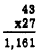Breaking the 27 into 20 + 7, we have 7 units times 43 plus 2 tens times 43, are follows:Since 7 units times 43 is 301 units, and 2 tens times 43 is 86 tens, we have the following:As long as the partial products are written in the correct columns, we can multiply beginning from either the left or the right of the multiplier. Thus, multiplying from the left, we have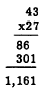Multiplication by a number having more places involves no new ideas. End Zeros The placement of partial products must be kept in mind when multiplying in problems involving end zeros, as in the following example: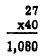We have 0 units times 27 plus 4 tens times 27, as follows: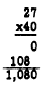The zero in the units place plays an important part in the reading of the final product. End zeros are often called "place holders" since their only function in the problem is to hold the digit positions which they occupy, thus helping to place the other digits in the problem correctly. The end zero in the foregoing problem can be accounted for very nicely, while at the same time placing the other digits correctly, by means of a shortcut. This consists of offsetting the 40 one place to the right and then simply bringing down the 0, without using it as a multiplier at all. The problem would appear as follows: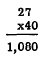If the problem involves a multiplier with more than one end 0, the multiplier is offset as many places to the right as there are end 0’s. For example, consider the following multiplication in which the multiplier, 300, has two end O’s:Notice that there are as many place-holding zeros at the end in the product as there are place-holding zeros in the multiplier and the multiplicand combined.Integrated Publishing, Inc. - A (SDVOSB) Service Disabled Veteran Owned Small Business